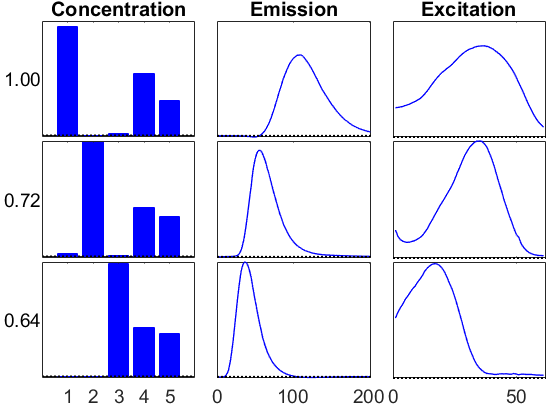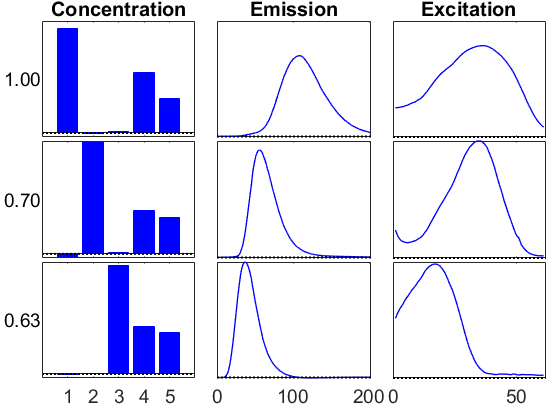# Alternating least squares for CANDECOMP/PARAFAC (CP) Decomposition

The function cp_als computes an estimate of the best rank-R CP model of a tensor X using the well-known alternating least-squares algorithm (see, e.g., Kolda and Bader, SIAM Review, 2009, for more information). The input X can be almost any type of tensor inclusing a tensor, sptensor, ktensor, or ttensor. The output CP model is a ktensor.

## Contents

We use the well-known amino acids data set from Andersson and Bro. It contains fluorescence measurements of 5 samples containing 3 amino acids: Tryptophan, Tyrosine, and Phenylalanine.Each amino acid corresponds to a rank-one component. The tensor is of size 5 x 51 x 201 from 5 samples, 51 excitations, and 201 emissions. Further details can be found here: http://www.models.life.ku.dk/Amino_Acid_fluo. Please cite the following paper for this data: Rasmus Bro, PARAFAC: Tutorial and applications, Chemometrics and Intelligent Laboratory Systems, 1997, 38, 149-171. This dataset can be found in the doc directory.

```load aminoacids
```

## Basic call to the method, specifying the data tensor and its rank

This uses a random initial guess. At each iteration, it reports the 'fit' which is defined as 1-(norm(X-M)/norm(X)) and is loosely the proportion of the data described by the CP model, i.e., a fit of 1 is perfect.

```rng('default') %<- Setting random seed for reproducibility of this script
M1 = cp_als(X,3); %<- Call the method
```
```CP_ALS:
Iter  1: f = 5.757392e-01 f-delta = 5.8e-01
Iter  2: f = 6.397722e-01 f-delta = 6.4e-02
Iter  3: f = 6.475932e-01 f-delta = 7.8e-03
Iter  4: f = 6.569483e-01 f-delta = 9.4e-03
Iter  5: f = 6.784483e-01 f-delta = 2.1e-02
Iter  6: f = 7.272329e-01 f-delta = 4.9e-02
Iter  7: f = 7.743007e-01 f-delta = 4.7e-02
Iter  8: f = 8.109037e-01 f-delta = 3.7e-02
Iter  9: f = 8.574394e-01 f-delta = 4.7e-02
Iter 10: f = 9.072207e-01 f-delta = 5.0e-02
Iter 11: f = 9.370083e-01 f-delta = 3.0e-02
Iter 12: f = 9.516441e-01 f-delta = 1.5e-02
Iter 13: f = 9.595934e-01 f-delta = 7.9e-03
Iter 14: f = 9.640126e-01 f-delta = 4.4e-03
Iter 15: f = 9.665412e-01 f-delta = 2.5e-03
Iter 16: f = 9.681171e-01 f-delta = 1.6e-03
Iter 17: f = 9.692201e-01 f-delta = 1.1e-03
Iter 18: f = 9.700730e-01 f-delta = 8.5e-04
Iter 19: f = 9.707752e-01 f-delta = 7.0e-04
Iter 20: f = 9.713716e-01 f-delta = 6.0e-04
Iter 21: f = 9.718846e-01 f-delta = 5.1e-04
Iter 22: f = 9.723272e-01 f-delta = 4.4e-04
Iter 23: f = 9.727090e-01 f-delta = 3.8e-04
Iter 24: f = 9.730376e-01 f-delta = 3.3e-04
Iter 25: f = 9.733198e-01 f-delta = 2.8e-04
Iter 26: f = 9.735616e-01 f-delta = 2.4e-04
Iter 27: f = 9.737684e-01 f-delta = 2.1e-04
Iter 28: f = 9.739449e-01 f-delta = 1.8e-04
Iter 29: f = 9.740954e-01 f-delta = 1.5e-04
Iter 30: f = 9.742235e-01 f-delta = 1.3e-04
Iter 31: f = 9.743326e-01 f-delta = 1.1e-04
Iter 32: f = 9.744253e-01 f-delta = 9.3e-05
Final f = 9.744253e-01
```

We typically can achieve a final fit of f = 0.97. The method stops when the change in the fit becomes less than the specified tolerance, which defaults to 1-e4.

## Visualize the results

Use the ktensor/viz function to visualize the results.

```vizopts = {'PlotCommands',{'bar','line','line'},...
'ModeTitles',{'Concentration','Emission','Excitation'},...
'BottomSpace',0.10,'HorzSpace',0.04,'Normalize',0};
info1 = viz(M1,'Figure',1,vizopts{:});
```## Run again with a different initial guess, output the initial guess.

This time we have two outputs. The first output is the solution as a ktensor. The second output is a cell array containing the initial guess. Since the first mode is not needed, it is omitted from the cell array.

```[M2bad,U2] = cp_als(X,3);
```
```CP_ALS:
Iter  1: f = 5.372277e-01 f-delta = 5.4e-01
Iter  2: f = 6.706563e-01 f-delta = 1.3e-01
Iter  3: f = 7.479181e-01 f-delta = 7.7e-02
Iter  4: f = 7.936023e-01 f-delta = 4.6e-02
Iter  5: f = 8.182495e-01 f-delta = 2.5e-02
Iter  6: f = 8.513821e-01 f-delta = 3.3e-02
Iter  7: f = 9.025108e-01 f-delta = 5.1e-02
Iter  8: f = 9.523589e-01 f-delta = 5.0e-02
Iter  9: f = 9.686955e-01 f-delta = 1.6e-02
Iter 10: f = 9.716873e-01 f-delta = 3.0e-03
Iter 11: f = 9.725830e-01 f-delta = 9.0e-04
Iter 12: f = 9.730243e-01 f-delta = 4.4e-04
Iter 13: f = 9.733194e-01 f-delta = 3.0e-04
Iter 14: f = 9.735495e-01 f-delta = 2.3e-04
Iter 15: f = 9.737412e-01 f-delta = 1.9e-04
Iter 16: f = 9.739052e-01 f-delta = 1.6e-04
Iter 17: f = 9.740470e-01 f-delta = 1.4e-04
Iter 18: f = 9.741698e-01 f-delta = 1.2e-04
Iter 19: f = 9.742764e-01 f-delta = 1.1e-04
Iter 20: f = 9.743687e-01 f-delta = 9.2e-05
Final f = 9.743687e-01
```

## Increase the maximium number of iterations

Note that the previous run kicked out at only 50 iterations before reaching the specified convegence tolerance. Let's increate the maximum number of iterations and try again, using the same initial guess.

```M2 = cp_als(X,3,'maxiters',100,'init',U2);
```
```CP_ALS:
Iter  1: f = 5.372277e-01 f-delta = 5.4e-01
Iter  2: f = 6.706563e-01 f-delta = 1.3e-01
Iter  3: f = 7.479181e-01 f-delta = 7.7e-02
Iter  4: f = 7.936023e-01 f-delta = 4.6e-02
Iter  5: f = 8.182495e-01 f-delta = 2.5e-02
Iter  6: f = 8.513821e-01 f-delta = 3.3e-02
Iter  7: f = 9.025108e-01 f-delta = 5.1e-02
Iter  8: f = 9.523589e-01 f-delta = 5.0e-02
Iter  9: f = 9.686955e-01 f-delta = 1.6e-02
Iter 10: f = 9.716873e-01 f-delta = 3.0e-03
Iter 11: f = 9.725830e-01 f-delta = 9.0e-04
Iter 12: f = 9.730243e-01 f-delta = 4.4e-04
Iter 13: f = 9.733194e-01 f-delta = 3.0e-04
Iter 14: f = 9.735495e-01 f-delta = 2.3e-04
Iter 15: f = 9.737412e-01 f-delta = 1.9e-04
Iter 16: f = 9.739052e-01 f-delta = 1.6e-04
Iter 17: f = 9.740470e-01 f-delta = 1.4e-04
Iter 18: f = 9.741698e-01 f-delta = 1.2e-04
Iter 19: f = 9.742764e-01 f-delta = 1.1e-04
Iter 20: f = 9.743687e-01 f-delta = 9.2e-05
Final f = 9.743687e-01
```

This solution looks more or less the same as the previous one.

```info2 = viz(M2,'Figure',2,vizopts{:});
```## Compare the two solutions

Use the ktensor/score function to compare the two solutions. A score of 1 indicates a perfect match. These are not exactly the same, but they are pretty close.

```score(M1,M2)
```
```ans =
0.9976
```

## Rerun with same initial guess

Using the same initial guess (and all other parameters) gives the exact same solution.

```M2alt = cp_als(X,3,'maxiters',100,'init',U2);
score(M2, M2alt) %<- Score of 1 indicates the same solution
```
```CP_ALS:
Iter  1: f = 5.372277e-01 f-delta = 5.4e-01
Iter  2: f = 6.706563e-01 f-delta = 1.3e-01
Iter  3: f = 7.479181e-01 f-delta = 7.7e-02
Iter  4: f = 7.936023e-01 f-delta = 4.6e-02
Iter  5: f = 8.182495e-01 f-delta = 2.5e-02
Iter  6: f = 8.513821e-01 f-delta = 3.3e-02
Iter  7: f = 9.025108e-01 f-delta = 5.1e-02
Iter  8: f = 9.523589e-01 f-delta = 5.0e-02
Iter  9: f = 9.686955e-01 f-delta = 1.6e-02
Iter 10: f = 9.716873e-01 f-delta = 3.0e-03
Iter 11: f = 9.725830e-01 f-delta = 9.0e-04
Iter 12: f = 9.730243e-01 f-delta = 4.4e-04
Iter 13: f = 9.733194e-01 f-delta = 3.0e-04
Iter 14: f = 9.735495e-01 f-delta = 2.3e-04
Iter 15: f = 9.737412e-01 f-delta = 1.9e-04
Iter 16: f = 9.739052e-01 f-delta = 1.6e-04
Iter 17: f = 9.740470e-01 f-delta = 1.4e-04
Iter 18: f = 9.741698e-01 f-delta = 1.2e-04
Iter 19: f = 9.742764e-01 f-delta = 1.1e-04
Iter 20: f = 9.743687e-01 f-delta = 9.2e-05
Final f = 9.743687e-01
ans =
1
```

## Changing the output frequency

Using the 'printitn' option to change the output frequency.

```M2alt2 = cp_als(X,3,'maxiters',100,'init',U2,'printitn',10);
```
```CP_ALS:
Iter 10: f = 9.716873e-01 f-delta = 3.0e-03
Iter 20: f = 9.743687e-01 f-delta = 9.2e-05
Final f = 9.743687e-01
```

## Suppress all output

Set 'printitn' to zero to suppress all output.

```M2alt3 = cp_als(X,3,'maxiters',100,'init',U2,'printitn',0); % <-No output
```

## Use HOSVD initial guess

Use the 'nvecs' option to use the leading mode-n singular vectors as the initial guess.

```M3 = cp_als(X,3,'init','nvecs','printitn',10);
```
```CP_ALS:
Iter 10: f = 9.334888e-01 f-delta = 3.5e-03
Iter 20: f = 9.604549e-01 f-delta = 1.9e-03
Iter 30: f = 9.712518e-01 f-delta = 5.8e-04
Iter 40: f = 9.741285e-01 f-delta = 1.4e-04
Iter 43: f = 9.744312e-01 f-delta = 8.6e-05
Final f = 9.744312e-01
```

Compare to the first solution using score, and see they are nearly the same because the score is close to 1.

```score(M1,M3)
```
```ans =
0.9859
```

## Change the order of the dimensions in CP

```[M4,~,info] = cp_als(X,3,'dimorder',[2 3 1],'init','nvecs','printitn',10);
score(M1,M4)
```
```CP_ALS:
Iter 10: f = 9.449957e-01 f-delta = 3.1e-03
Iter 20: f = 9.657394e-01 f-delta = 1.3e-03
Iter 30: f = 9.727566e-01 f-delta = 3.5e-04
Iter 39: f = 9.743928e-01 f-delta = 9.2e-05
Final f = 9.743928e-01
ans =
0.9855
```

In the last example, we also collected the third output argument which has some extra information in it. The field info.iters has the total number of iterations. The field info.params has the information used to run the method. Unless the initialization method is 'random', passing the parameters back to the method will yield the exact same results.

```M4alt = cp_als(X,3,info.params);
score(M4,M4alt)
```
```CP_ALS:
Iter 10: f = 9.449957e-01 f-delta = 3.1e-03
Iter 20: f = 9.657394e-01 f-delta = 1.3e-03
Iter 30: f = 9.727566e-01 f-delta = 3.5e-04
Iter 39: f = 9.743928e-01 f-delta = 9.2e-05
Final f = 9.743928e-01
ans =
1
```

## Change the tolerance

It's also possible to loosen or tighten the tolerance on the change in the fit. You may need to increase the number of iterations for it to converge.

```M5 = cp_als(X,3,'init','nvecs','tol',1e-6,'maxiters',1000,'printitn',10);
```
```CP_ALS:
Iter 10: f = 9.334888e-01 f-delta = 3.5e-03
Iter 20: f = 9.604549e-01 f-delta = 1.9e-03
Iter 30: f = 9.712518e-01 f-delta = 5.8e-04
Iter 40: f = 9.741285e-01 f-delta = 1.4e-04
Iter 50: f = 9.747733e-01 f-delta = 2.9e-05
Iter 60: f = 9.749128e-01 f-delta = 6.4e-06
Iter 70: f = 9.749430e-01 f-delta = 1.4e-06
Iter 73: f = 9.749461e-01 f-delta = 8.8e-07
Final f = 9.749461e-01
```

## Control sign ambiguity of factor matrices

The default behavior of cp_als is to make a call to fixsigns to fix the sign ambiguity of the factor matrices. You can turn off this behavior by passing the 'fixsigns' parameter value of false when calling cp_als.

```X = ktensor([1;1], {[1, 1; 1, -10],[1, 1; 1, -10]});
M = cp_als(X, 2, 'printitn', 0, 'init', X.U) % <-default behavior, fixsigns called
M = cp_als(X, 2, 'printitn', 0, 'init', X.U, 'fixsigns', false) % <-fixsigns not called
```
```M is a ktensor of size 2 x 2
M.lambda =
101.0000    2.0000
M.U{1} =
-0.0995    0.7071
0.9950    0.7071
M.U{2} =
-0.0995    0.7071
0.9950    0.7071
M is a ktensor of size 2 x 2
M.lambda =
101.0000    2.0000
M.U{1} =
0.0995    0.7071
-0.9950    0.7071
M.U{2} =
0.0995    0.7071
-0.9950    0.7071
```

## Recommendations

• Run multiple times with different guesses and select the solution with the best fit.
• Try different ranks and choose the solution that is the best descriptor for your data based on the combination of the fit and the interpretaton of the factors, e.g., by visualizing the results.